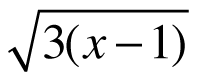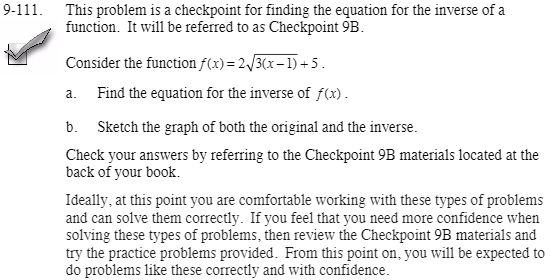### Home > CCA2 > Chapter Ch9 > Lesson 9.3.3 > Problem9-111

9-111.
1.9-111. This problem is a checkpoint for finding the equation for the inverse of a function. It will be referred to as Checkpoint 9B. Homework Help ✎

2. Consider the function f(x) =+ 5 .

1. Find the equation for the inverse of f(x).

2. Sketch the graph of both the original and the inverse.